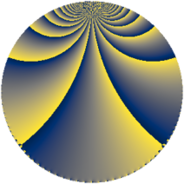# Properties

 Label 684.3.bLevel $684$ Weight $3$ Character orbit 684.b Rep. character $\chi_{684}(683,\cdot)$ Character field $\Q$ Dimension $80$ Newform subspaces $1$ Sturm bound $360$ Trace bound $0$

# Related objects

## Defining parameters

 Level: $$N$$ $$=$$ $$684 = 2^{2} \cdot 3^{2} \cdot 19$$ Weight: $$k$$ $$=$$ $$3$$ Character orbit: $$[\chi]$$ $$=$$ 684.b (of order $$2$$ and degree $$1$$) Character conductor: $$\operatorname{cond}(\chi)$$ $$=$$ $$228$$ Character field: $$\Q$$ Newform subspaces: $$1$$ Sturm bound: $$360$$ Trace bound: $$0$$

## Dimensions

The following table gives the dimensions of various subspaces of $$M_{3}(684, [\chi])$$.

Total New Old
Modular forms 248 80 168
Cusp forms 232 80 152
Eisenstein series 16 0 16

## Trace form

 $$80 q - 8 q^{4} + O(q^{10})$$ $$80 q - 8 q^{4} - 56 q^{16} - 400 q^{25} - 464 q^{49} - 272 q^{58} - 352 q^{61} - 200 q^{64} + 480 q^{73} + 152 q^{76} + 32 q^{82} + 704 q^{85} + O(q^{100})$$

## Decomposition of $$S_{3}^{\mathrm{new}}(684, [\chi])$$ into newform subspaces

Label Dim $A$ Field CM Traces $q$-expansion
$a_{2}$ $a_{3}$ $a_{5}$ $a_{7}$
684.3.b.a $80$ $18.638$ None $$0$$ $$0$$ $$0$$ $$0$$

## Decomposition of $$S_{3}^{\mathrm{old}}(684, [\chi])$$ into lower level spaces

$$S_{3}^{\mathrm{old}}(684, [\chi]) \cong$$ $$S_{3}^{\mathrm{new}}(228, [\chi])$$$$^{\oplus 2}$$